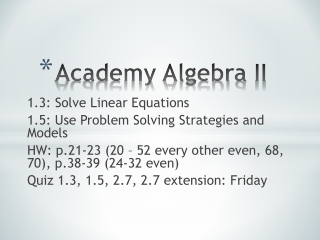DownloadDownload PresentationAcademy Algebra II

# Academy Algebra II

Download Presentation## Academy Algebra II

- - - - - - - - - - - - - - - - - - - - - - - - - - - E N D - - - - - - - - - - - - - - - - - - - - - - - - - - -
##### Presentation Transcript

1. Academy Algebra II 1.3: Solve Linear Equations 1.5: Use Problem Solving Strategies and Models HW: p.21-23 (20 – 52 every other even, 68, 70), p.38-39 (24-32 even) Quiz 1.3, 1.5, 2.7, 2.7 extension: Friday

2. 1.3 Do Now: Solve. 1.) 2.) 12(r + 3) = 2(r + 5) – 3r

3. 3.) The bill for the repair of your bicycle was \$180. The cost of parts was \$105. The cost of labor was \$25 per hour. How many hours did the repair work take? p.23 #69

4. 4.) You have two summer jobs. In the first job, you work 25 hours per week and earn \$7.75 per hour. In the second job, you earn \$6.25 per hour and can work as many hours you want. You want to earn \$250 per week. Write an equation and find how many hours you must work at the second job. p.23 #71

5. 5.) 2(b + 3) = 4b – 2 6.) 2x – 3 = 6x + 25 7.) Solve the equation. Check your solution

6. 8.) 0.6x + 0.5 = 2.9 9.) 10.) 3.8w + 3.2 = 2.3(w + 4) Solve the equation.

7. p.35 Example 3: 11.) You are hanging 4 championship banners on a wall in your school’s gym. The banners are 8 feet wide and the wall is 62 feet long. There should an equal amount of space between the ends of the wall and the banners, and between each pair of banners. How far apart should the banners be placed?

8. 12.) You have a piece of wood that is 72 inches long. You cut the wood into three pieces. The second piece is 6 inches longer than the first piece. The third piece is 6 inches longer than the second piece. Write and solve an equation to find the lengths of the three pieces. p.39 #29

9. 13.) A moving company weighs 20 boxes you have packed that contain either books or clothes and says the total weight is 404 pounds. You know that a box of books weighs 40 pounds and a box of clothes weighs 7 pounds. Write and solve an equation to find how many boxes of books ad how many boxes of clothes you packed. p.39 #31